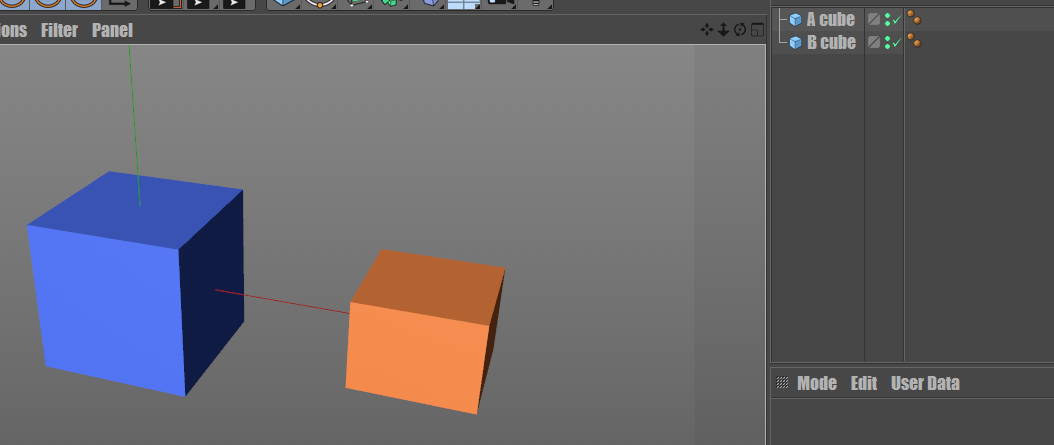# SOLVEDBeginner：How to set A cube as the child of B cube by python?

Hi~I recently meet the problem to batch move objects with the same offset distance, however, to set position respectively can be a disaster and a little fool, so I want to make them as children of a Null object that I can move all of them with just move Null object.

To let other potential beginners better pick up and understand, let me make 2 cubes as an easy example, so how to set B cube as the child of A cube by python? what if two B cubes or more?``````import c4d
A_cube=c4d.BaseObject(c4d.Ocube)
B_cube=c4d.BaseObject(c4d.Ocube)
#?????unknow how to set children ???

doc.InsertObject(B_cube)

``````

Thanks!

TO inserts cube as a child of another one, call BaseList2D.InsertUnder Then here you can call BaseObject.SetRelPos To set the relative position. The relative position is relative to the parent so this way you can easily have the same distance between multiple objects.

``````import c4d

# Main function
def main():
# Creates 3 cubes in memory
aCube = c4d.BaseObject(c4d.Ocube)
bCube = c4d.BaseObject(c4d.Ocube)
cCube = c4d.BaseObject(c4d.Ocube)

# Inserts C under B, and B under A
cCube.InsertUnder(bCube)
bCube.InsertUnder(aCube)

# Defines the relative position of B and C
bCube.SetRelPos(c4d.Vector(250, 0, 0))
cCube.SetRelPos(c4d.Vector(250, 0, 0))

# Inserts a and all its children into the current document
doc.InsertObject(aCube)

# Pushes an update event to Cinema 4D

# Execute main()
if __name__=='__main__':
main()
``````

Finally, while it's in C++, all the knowledge is applicable in python so I warmly suggest you to read the GeListNode Manual.

If you have any questions, let me know.
Cheers,
Maxime.